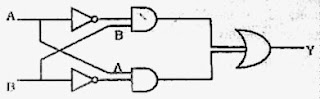## Wednesday, February 03, 2010

### Kerala Engineering and Medical Entrance Examination (KEAM) 2009 Questions on Digital Circuits

The following question appeared in Kerala Engineering Entrance 2009 Examination question paper:

The truth table for the following logic circuit isThe top AND gate gets inputs (NOT A) and B. The bottom AND gate gets inputs A and (NOT B). The outputs of the two AND gates are respectively [(NOT A) AND B] and [A AND (NOT B)]. These are the inputs for the final OR gate. The output of the final OR gate is [(NOT A) AND B] OR [A AND (NOT B)]. This is the Boolean expression for the XOR gate (exclusive OR gate, also termed EXOR gate). The truth table for the XOR gate is given in option (A).

Even if you don’t know the XOR gate and its truth table, you can substitute values 0 and 1 for the inputs A and B and find the output in each trial. You can easily arrive at the answer given in option (A).

The following question appeared in Kerala Medical Entrance 2009 Examination question paper:

If the two inputs of a NAND gate are shorted, the gate is equivalent to

(a) XOR

(b) OR

(c) NOR

(d) NOT

(e) AND

If both inputs of a two input NAND gate are shorted, both inputs get the same signal. Because of the NOT operation at the output side of the NAND, the output is the complement of the input. The circuit therefore behaves as a NOT gate [Option (d)].

#### 1 comment:

1.HEy its nice one on truth table ..but you can see some very good stuff for Physics for Engineering Entrance on www.iitbanda.com.
Anything related to IIT JEE & AIEEE visit www.iitbanda.com